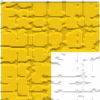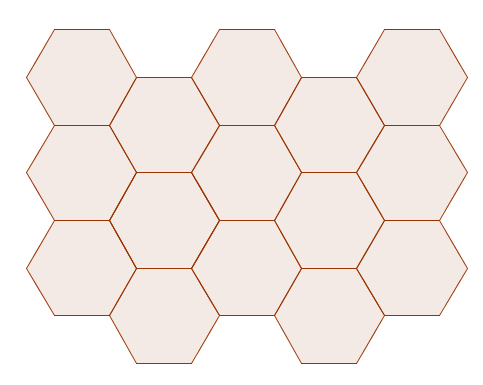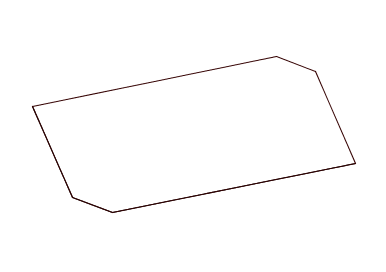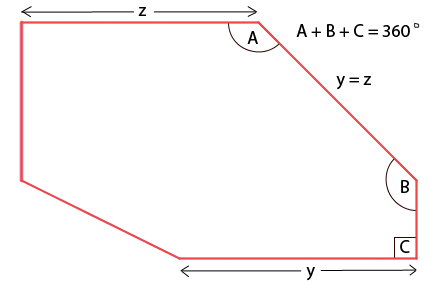You may also likeTriominoes

A triomino is a flat L shape made from 3 square tiles. A chess board is marked into squares the same size as the tiles and just one square, anywhere on the board, is coloured red. Can you cover the board with trionimoes so that only the square is exposed?LOGO Challenge 5 - Patch

Using LOGO, can you construct elegant procedures that will draw this family of 'floor coverings'?LOGO Challenge - Triangles-squares-stars

Can you recreate these designs? What are the basic units? What movement is required between each unit? Some elegant use of procedures will help - variables not essential.

Tessellating Hexagons

Age 11 to 14Challenge Level

This problem follows on from some of the ideas in Tessellating Triangles and Tessellating Quadrilaterals. It was inspired by a problem in "Tiles and Tiling", an activity book published by the Association of Teachers of Mathematics.

Here is a tessellation of regular hexagons:Can you explain why regular hexagons tessellate?

What about a hexagon where each pair of opposite sides is parallel, and opposite sides are the same length, but different pairs of sides are not the same length?You can print off some square dotty paper, or some isometric dotty paper, and try drawing hexagons of this form on it. You could also draw some hexagons using this interactive. Can you tessellate them?

Do all hexagons of this form tessellate? How do you know?

Now let's consider hexagons with three adjacent angles which add up to $360^{\circ}$, sandwiched by two sides of equal length, as in the diagram below:Is it true that any hexagon of this form will tessellate? How do you know?
You could start by convincing yourself that sides $y$ and $z$ are parallel...